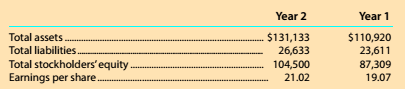Chapter 8, Problem 8.10.3MBA

Chapter
Section
Textbook Problem

Debt and price-earnings ratios Alphabet (formerly known as Google) (GOOG) is a technology company that offers users Internet search and e-mail services. Google also developed the Android operating system for use with cell phones and other mobile devices. The following data (in millions) were adapted from a recent financial statement of Alphabet.Compute the ratio of liabilities to stockholders' equity. Round to one decimal place.

To determine

Concept Introduction:

Debt Ratio:

Debt ratio is the relationship between the Total liabilities and Total Assets of a corporation. Debt ratio shows the part of assets financed by debts. It is calculated by dividing total liabilities by total assets. The formula of debt ratio is as follows:

Debt Ratio = Total LiabilitiesTotal Assets

To Calculate:

The Ratios of Liabilities to Stockholder's Equity for year 1 and 2

Explanation

The Ratios of Liabilities to Stockholder's Equity for year 1 and 2 are calculated as follows:

 \$ in Millions Year 1 Year 2 Stockholder's Equity (A)

Still sussing out bartleby?

Check out a sample textbook solution.

See a sample solution

The Solution to Your Study Problems

Bartleby provides explanations to thousands of textbook problems written by our experts, many with advanced degrees!

Get Started

Explain how a trial balance and a balance sheet differ.

College Accounting (Book Only): A Career Approach

Looking at the income statement, what are the company's most recent sales and net income? Over the past several...

Fundamentals of Financial Management, Concise Edition (with Thomson ONE - Business School Edition, 1 term (6 months) Printed Access Card) (MindTap Course List)

Should an economic model describe reality exactly?

Principles of Microeconomics (MindTap Course List)